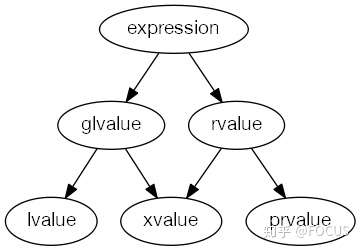# 现代 C++：右值引用、移动语意、完美转发

2021-09-25 258点热度 0人点赞 0条评论
转载From https://zhuanlan.zhihu.com/p/150039719 by FOCUS


## 移动语义

1. v2 = v1 调用的是拷贝赋值操作符，v2 复制了 v1 的内容 —— 复制语义。
2. v3 = std::move(v1) 调用的是移动赋值操作符，将 v1 的内容移动给 v3 —— 移动语义。
  std::vector<int> v1{1, 2, 3, 4, 5};
std::vector<int> v2;
std::vector<int> v3;

v2 = v1;
std::cout << v1.size() << std::endl;  // 输出 5
std::cout << v2.size() << std::endl;  // 输出 5

v3 = std::move(v1); // move
std::cout << v1.size() << std::endl;  // 输出0
std::cout << v3.size() << std::endl;  // 输出 5


#include
#include
#include

class Foo {
public:
// 默认构造函数
Foo() { std::cout << "Default Constructor: " << Info() << std::endl; }

// 自定义构造函数
Foo(const std::string& s, const std::vector& v) : s_(s), v_(v) {
std::cout << "User-Defined Constructor: " << Info() << std::endl;
}

// 析构函数
~Foo() { std::cout << "Destructor: " << Info() << std::endl; }

// 拷贝构造函数
Foo(const Foo& f) : s_(f.s_), v_(f.v_) {
std::cout << "Copy Constructor: " << Info() << std::endl;
}

// 拷贝赋值操作符
Foo& operator=(const Foo& f) {
s_ = f.s_;
v_ = f.v_;
std::cout << "Copy Assignment: " << Info() << std::endl;
return *this;
}

// 移动构造函数
Foo(Foo&& f) : s_(std::move(f.s_)), v_(std::move(f.v_)) {
std::cout << "Move Constructor: " << Info() << std::endl;
}

// 移动赋值操作符
Foo& operator=(Foo&& f) {
s_ = std::move(f.s_);
v_ = std::move(f.v_);
std::cout << "Move Assignment: " << Info() << std::endl;
return *this;
}

std::string Info() {
return "{" + (s_.empty() ? "'empty'" : s_) + ", " +
std::to_string(v_.size()) + "}";
}

private:
std::string s_;
std::vector v_;
};

int main() {
std::vector v(1024);

std::cout << "================ Copy =======================" << std::endl;
Foo cf1("hello", v);
Foo cf2(cf1);  // 调用拷贝构造函数
Foo cf3;
cf3 = cf2;  // 调用拷贝赋值操作符

std::cout << "================ Move =========================" << std::endl;
Foo f1("hello", v);
Foo f2(std::move(f1));  // 调用移动构造函数
Foo f3;
f3 = std::move(f2);  // 调用移动赋值操作符
return 0;
}


• 拷贝语意：拷贝构造函数 Foo(const Foo&) 、拷贝赋值操作符 Foo& operator=(const Foo&)
• 移动语意：移动构造函数 Foo(Foo&&) 、移动赋值操作符 Foo& operator=(Foo&&)

Foo GetFoo() {
return Foo("GetFoo", std::vector<int>(11));
}
....
Foo f3("world", v3);
....
f3 = GetFoo(); // GetFoo 返回的是一个右值，调用移动赋值操作符


## 完美转发

C++ 通过了一个叫 std::forward 的函数模板来实现完美转发。这里直接使用 Effective Modern C++ 中的例子作为说明。在前面的例子上，我们增加如下的代码：

// 接受一个 const 左值引用
void Process(const Foo& f) {
std::cout << "lvalue reference" << std::endl;
// ...
}

// 接受一个右值引用
void Process(Foo&& f) {
std::cout << "rvalue reference" << std::endl;
// ...
}

template <typename T>
void LogAndProcessNotForward(T&& a) {
std::cout << a.Info() << std::endl;
Process(a);
}

template <typename T>
void LogAndProcessWithForward(T&& a) {
std::cout << a.Info() << std::endl;
Process(std::forward<T>(a));
}

LogAndProcessNotForward(f3);                         // 输出 lvalue reference
LogAndProcessNotForward(std::move(f3));  // 输出 lvalue reference

LogAndProcessWithForward(f3);                        // 输出 lvalue reference
LogAndProcessWithForward(std::move(f3));  // 输出 rvalue reference

• LogAndProcessNotForward(f3);LogAndProcessWithForward(f3); 都输出 "lvalue reference"，这一点都不意外，因为 f3 本来就是一个左值。
• LogAndProcessNotForward(std::move(f3)); 输出 "lvalue reference" 是因为虽然参数 a 绑定到一个右值，但是参数 a 本身是一个左值。
• LogAndProcessWithForward(std::move(f3)); 使用了 std::forward 对参数进行转发，std::forward 的作用就是：当参数是绑定到一个右值时，就将参数转换成一个右值。

## 左值？右值？

1. has identity? —— 是否有唯一标识，比如地址、指针。有唯一标识的表达式在 C++ 中被称为 glvalue（generalized lvalue）。
2. can be moved from? —— 是否可以安全地移动（编译器）。可以安全地移动的表达式在 C++ 中被成为 rvalue。

1. has identity and cannot be moved from - 这类表达式在 C++ 中被称为 lvalue。
2. has identity and can be moved from - 这类表达式在 C++ 中被成为 xvalue（expiring value）。
3. does not have identity and can be moved from - 这类表达式在 C++ 中被成为 prvalue（pure rvalue）。
4. does not have identity and cannot be moved -C++ 中不存在这类表达式。

1. 可以移动的值都叫 rvalue，包括 xvalue 和 prvalue。
2. 有唯一标识的值都叫 glvalue，包括 lvalue 和 xvalue。
3. std::move 的作用就是将一个 lvalue 转换成 xvalue。## 参考文档kerbal